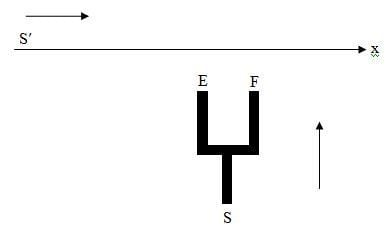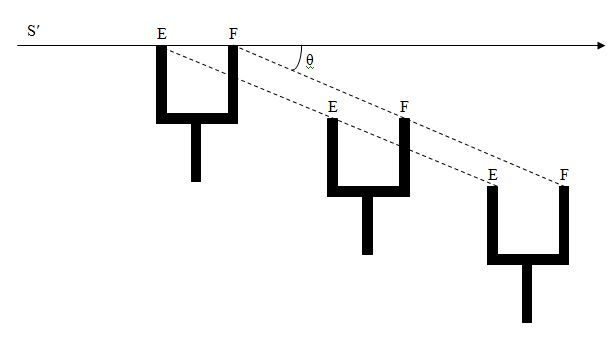# Relativity of Simultaneity Issue

Hi,

Consider an observer S pushing a fork towards a material x-axis as seen in the figure belowAccording to this observer E and F cut x-axis simultaneously.

Now consider an observer S' moving along x-axis with a constant relativistic speed. It is very easy, using Lorentz transformation, to show that E and F will NOT cut x-axis simultaneously (according to S')

How is it possible that E and F will not cut x-axis simultaneously with respect to S' knowing that the none of the arms of the fork will undergo any length variation because they move perpendicular to the motion of S'?

Doc Al
Mentor
How is it possible that E and F will not cut x-axis simultaneously with respect to S' knowing that the none of the arms of the fork will undergo any length variation because they move perpendicular to the motion of S'?
From the view of S', the fork is moving at an angle with respect to the x' axis.

•1 person
From the view of S', the fork is moving at an angle with respect to the x' axis.

Hmm, yes this is right, I have missed it; it is clear now that according to S', F will hit x' axis first.
Thank you.

Sorry, I am confused again.

Even if the fork moves at an angle with respect to S', E and F would still hit x' axis simultaneously!PAllen
Let's take away the complexity of arms and angles, and replace the fork by just the ends of its two arms. Let's also make the unprimed x axis 'physical' as follows:

- every tenth of a second, a chain lights flash simultaneous all along a section of the x axis; each tenth of a second, the color of flashes changes.

Now suppose the fork ends are arranged to hit when the light flash green. Then both S and S' see the fork ends smashing a green flashing light. To S, these lights flashed simultaneously. To S' they do not fire simultaneously. No paradox. I hope this is clear.

[edit: I guess, to complete the mental picture, angles are useful. In S', not only is the direction of motion of the fork at an angle to x, also the fork is tilted slightly. ]

Last edited:
Let's take away the complexity of arms and angles, and replace the fork by just the ends of its two arms. Let's also make the unprimed x axis 'physical' as follows:

- every tenth of a second, a chain lights flash simultaneous all along a section of the x axis; each tenth of a second, the color of flashes changes.

Now suppose the fork ends are arranged to hit when the light flash green. Then both S and S' see the fork ends smashing a green flashing light. To S, these lights flashed simultaneously. To S' they do not fire simultaneously. No paradox. I hope this is clear.

[edit: I guess, to complete the mental picture, angles are useful. In S', not only is the direction of motion of the fork at an angle to x, also the fork is tilted slightly. ]

Thanks to your useful reply. Actually my main problem is the mental picture. I realize that the only way for this to happen is that the fork should rotate slightly (according to S'), but I can not see any relativistic reason for this rotation.

[edit: I guess, to complete the mental picture, angles are useful. In S', not only is the direction of motion of the fork at an angle to x, also the fork is tilted slightly. ]

Slightly more accurately, the fork is skewed or sheared in S' as per the attached diagram, but the handle and tines of the fork are still parallel to their original orientation in S. (i.e. it is not strictly a rotation of the fork as a whole, but more of a deformation of its shape.) Note that 'shape change' is quite normal in relativity. Since length contraction only occurs parallel to the axis of motion a square in S becomes a rectangle in S'. In some situations, rotation also occurs. (e.g. if we look at the cross piece of the fork in isolation.) Look up Thomas precession.

#### Attachments

Last edited:
•1 person
PAllen
Slightly more accurately, the fork is skewed or sheared in S' as per the attached diagram, but the handle and tines of the fork are still parallel to their original orientation in S. (i.e. it is not strictly a rotation of the fork as a whole, but more of a deformation of its shape.) Note that 'shape change' is quite normal in relativity. Since length contraction only occurs parallel to the axis of motion a square in S becomes a rectangle in S'. In some situations rotation also occurs. Look up Thomas precession.

Yes, this is more accurate wording. Nice picture.

Doc Al
Mentor
Sorry, I am confused again.

Even if the fork moves at an angle with respect to S', E and F would still hit x' axis simultaneously!

View attachment 61515
When I said it appears to be moving at an angle, I meant that it would appear to be skewed at an angle, not just moving along a diagonal. Sorry for not being clearer.

Thanks to PAllen and yuiop for clarifying and to yuiop for the nice diagram.

•1 person
But why the fork is going to skew?

PAllen
But why the fork is going to skew?

Here's an answer you won't like: because of the relativity of simultaneity. Consider the two fork ends pass a reference line at the same time in S. Then, in S', one leg of the fork crosses that line earlier. Therefore the fork is skewed in S'. You can take this back to the 'origin' of the fork: imagine it being extruded in S. If S extrudes the ends of the fork simultaneously, then S' say one leg was extruded earlier and continues to lead the other leg.

Or just do a Lorentz transform of some world lines defining the fork.

As yuiop noted earlier, this sort of shape change, rotation etc. are routine for relativity due to simultaneity differences.

•1 person
OK

But how can we know whether the fork is rotated or skewed, since rotation can also assure that E and F will not x' axis simultaneously?

Dale
Mentor
2021 Award
But how can we know whether the fork is rotated or skewed, since rotation can also assure that E and F will not x' axis simultaneously?
To do that you need to do the Lorentz transform.

To do that you need to do the Lorentz transform.

So we need to apply transformation along both x and y axes. Is it true to apply Lorentz transformation separately along x and y? Or are there other planar transforms (Like position vectors)?

PAllen
So we need to apply transformation along both x and y axes. Is it true to apply Lorentz transformation separately along x and y? Or are there other planar transforms (Like position vectors)?

The standard Lorentz transform involves t,x,y,z. It's just that for motion along x, you have y'=y, z'=z. However, the nuance here is that if you transform a few events representing key parts of the fork, you end up with something useless in the primed frame. The moving observer is not interested in: (the end of the left arm was here at t'=1, and the right arm was there at t'=2). The prime frame wants a set of defining points all at the same t' - that shows the shape the prime frame considers the fork to have.

To get this, you need to represent a few key points of the fork by world lines. Thus, in the unprimed frames it might be: (note: a is just a parameter for the world lines)

left arm tip: (t,x,y,z) = (a,1,0,0)
right arm tip: (t,x,y,z)=(a,2,0,0)
left arm base: (t,x,y,z)=(a,1,-1,0)
right arm base: (t,x,y,z)=(a,2,-1,0)

Transform these parametrized events just as if the were single coordinates. Then solve each transformed expression so that t' is the same (e.g. 0) for all four world lines. This will, in general involve a different value of 'a' for each defining point - because the prime frame disagrees with the unprimed frame about which points in the history of each piece of the fork are simultaneous.

•1 person
Dale
Mentor
2021 Award
So we need to apply transformation along both x and y axes. Is it true to apply Lorentz transformation separately along x and y? Or are there other planar transforms (Like position vectors)?
There are three frames involved here. There is the S frame, the S' frame, and the rest frame of the fork. The S' frame is related to the S frame by a Lorentz transform in x. The S frame is related to the rest frame of the fork by a Lorentz transform in y. So if you want to transform from the rest frame of the fork to the S' frame then yes, you need to transform in both y and x.

Of course, an alternative is to express the worldline of the fork in the S frame and then simply perform the transform to the S' frame. Either way you should get the same answer.

PAllen
Oh, right, I forgot the fork was supposed to be moving in S. In that case, I would just express what is assumed about the fork in S (Dalespam's second suggestion. Then you just have (for example):

left arm tip: (t,x,y,z) = (a,1,a/2,0)
right arm tip: (t,x,y,z)=(a,2,a/2,0)
left arm base: (t,x,y,z)=(a,1,a/2 - 1,0)
right arm base: (t,x,y,z)=(a,2,a/2 - 1,0)

(of course I'm using c=1 convention)

Last edited:

r'=r+(γ-1)(r.n)nvt

Can I apply it here to find r', then I relate x' and y' to find the trajectory of each of the points E and F?

PAllen

r'=r+(γ-1)(r.n)nvt

Can I apply it here to find r', then I relate x' and y' to find the trajectory of each of the points E and F?

Even assuming its applicable, it doesn't help deal with the key issue in seeing how shape changes between frames. For that, you must find a set of events on each world line that are simultaneous in new frame, and this will not be the transform of a set of events simultaneous in the original frame.

Really, it should be very straightforward for you to apply pure x direction Lorentz to:

left arm tip: (t,x,y,z) = (a,1,a/2,0)
right arm tip: (t,x,y,z)=(a,2,a/2,0)
left arm base: (t,x,y,z)=(a,1,a/2 - 1,0)
right arm base: (t,x,y,z)=(a,2,a/2 - 1,0)

then solve for t' = 0 for each world line, giving you an 'a' value for each. Use that to compute an x',y' for each world line at common t' times. Voila, you have the fork shape in the primed frame.

•1 person
PAllen
As a check for any work you may try, using the sample in my prior post, c=1 as noted, then if β,γ describe the x motion in S', the coordinates of a t' = 0 snapshot of the fork are:

left arm tip: x' = γ (1-β^2), y'=β/2
right arm tip: x' = 2γ(1-β^2),y'=β
left arm base: x'=γ(1-β^2), y'=β/2-1
right arem base: x' = 2γ(1-β^2),y'=β-1

For β=.6,γ=1.25

you get in the above order:

(.8,.3),(1.6,.6),(.8,-.7),(1.6,-.4)

showing, if you plot them, compression of distance between fork arms, no change in length of fork arms, no change in orientation of fork arms, but a skew of .3.

(Note: I've reversed the direction of S' compared to your drawing. I assume you can figure what signs to change to get the direction you want).

•1 person
Thank you all.
I have found the equation of the trajectory of each of the five vertices of the fork with respect to S'. The result is that all of them are parallel straight lines. It becomes clear that the fork is skewed and not rotated.